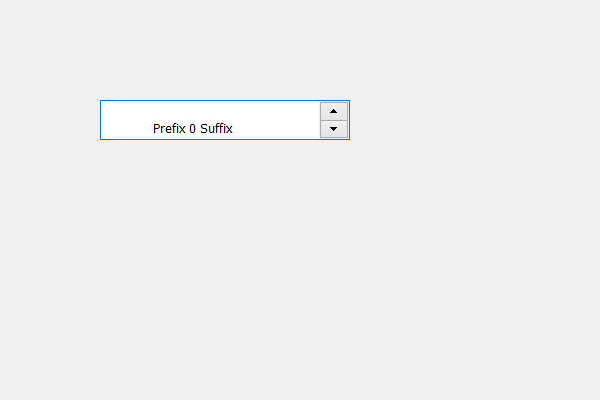# PyQt5 QSpinBox – Setting margin

• Last Updated : 19 May, 2020

In this article we will see how we can set margin to the spin box, by default there is no margin set to the spin box i.e their margin values are zero. Although we can change that, setting margin to the spin box will not display any change in the spin box due to overriding take place by the widget to maintain its structure. But in order to set margin to the spin box we have set margin to the spin box’s line edit object by which we can display the margin in spinbox.

In order to do this we use setContentsMargins method.

Syntax : line_edit.setContentsMargins(left, top, right, bottom)
Here line_edit is the QLineEdit object of the spin box

Argument : It takes four integer as argument

Return : It returns None

Below is the implementation

 `# importing libraries``from` `PyQt5.QtWidgets ``import` `*` `from` `PyQt5 ``import` `QtCore, QtGui``from` `PyQt5.QtGui ``import` `*` `from` `PyQt5.QtCore ``import` `*` `import` `sys`` ` ` ` `class` `Window(QMainWindow):`` ` `    ``def` `__init__(``self``):``        ``super``().__init__()`` ` `        ``# setting title``        ``self``.setWindowTitle(``"Python "``)`` ` `        ``# setting geometry``        ``self``.setGeometry(``100``, ``100``, ``600``, ``400``)`` ` `        ``# calling method``        ``self``.UiComponents()`` ` `        ``# showing all the widgets``        ``self``.show()`` ` `        ``# method for widgets``    ``def` `UiComponents(``self``):``        ``# creating spin box``        ``self``.spin ``=` `QSpinBox(``self``)`` ` `        ``# setting geometry to spin box``        ``self``.spin.setGeometry(``100``, ``100``, ``250``, ``40``)`` ` `        ``# setting range to the spin box``        ``self``.spin.setRange(``0``, ``999999``)`` ` `        ``# setting prefix to spin``        ``self``.spin.setPrefix(``"Prefix "``)`` ` `        ``# setting suffix to spin``        ``self``.spin.setSuffix(``" Suffix"``)`` ` `        ``# getting line edit object``        ``line_edit ``=` `self``.spin.lineEdit()`` ` `        ``# setting margin to the line edit``        ``line_edit.setContentsMargins(``50``, ``20``, ``5``, ``5``)`` ` `         ` ` ` `# create pyqt5 app``App ``=` `QApplication(sys.argv)`` ` `# create the instance of our Window``window ``=` `Window()``window.spin.setFocus()``# start the app``sys.exit(App.``exec``())`

Output :My Personal Notes arrow_drop_up